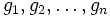# Tour:Multiplication table of a finite group

PREVIOUS: Introduction four (beginners)| UP: Introduction four (beginners)| NEXT: Isomorphism of groups
General instructions for the tour | Pedagogical notes for the tour | Pedagogical notes for this part
WHAT YOU NEED TO DO:
• Understand the definition of multiplication table of a finite group given below
• Understand the examples, and achieve comfort with the idea of using a multiplication table to multiply two elements of a group
• Read and understand the statements about how commutativity, associativity, identity elements and inverses affect the appearance of the multiplication table.

## Definition

### Definition with symbols

Let$G$ be a finite group of order$n$. Then, the multiplication table of$G$ is a$n \times n$ matrix described as follows:

• Label the elements of$G$ as$g_1,g_2,\ldots,g_n$ such that$g_1$ is the identity element.
• Now, in the$(i,j)^{th}$ entry of the matrix, put the element$g_ig_j$

Note that under this definition, the multiplication table is an$n \times n$ matrix with entries drawn from$G$. We can, however, change this to a$n \times n$ matrix with entries drawn from$1,2,\ldots,n$ by simply replacing$g \in G$ by the$k$ for which$g = g_k$.

The latter interpretation makes the multiplication table into a purely combinatorial object. We shall use this latter interpretation.

Clearly, the multiplication table determines the group uniquely.

We can also construct the multiplication table of any finite magma in the same way.

### Important note on commutativity

In a non-Abelian group, interchanging the role of rows and columns changes the multiplication table. Thus, we need to follow a convention (the convention here is that the row element is multiplied on the left and the column element is on the right).

For an Abelian group, the group operation is more typically termed addition, and the multiplication table is thus termed an addition table.

## Examples

### The trivial group

Here's how the multiplication table of the trivial group looks:

Element$e$ (identity element)$e$$e$

### A group of order two

Element$e$ (identity element)$x$ (non-identity element)$e$$e$$x$$x$$x$$e$

### A non-Abelian group of order six

Below is the multiplication table of a non-Abelian group of order six. This is actually the group of permutations on three letters (following the cycle decomposition notation).

## Properties

### Latin square

The multiplication table of a finite group is a Latin square, when we label the entries$1,2,\ldots,n$. In other words, every row contains each element exactly once and every column contains each element exactly once.

In fact, the multiplication table of a finite magma is a latin square if and only if that magma is a quasigroup. Groups are indeed quasigroups.

### Identity element

If we choose the label$1$ for the identity element, the first row and first column are both$1,2,\dots,n$.

### Commutativity yields symmetric Latin square

The multiplication table of a finite group (and more generally, of any finite magma) is a symmetric matrix if and only if the binary operation is commutative. In the case of groups, this boils down to saying that the group is Abelian.

### How inverses affect the Latin square

The existence of two-sided inverses means that, even if the Latin square is not symmetric, it is symmetric with respect to the identity element. In other words, if the$(i,j)^{th}$ entry is the identity element, then so is the$(j,i)^{th}$ entry.

### How associativity affects the Latin square

Not every Latin square arises from a group, even if the first row and first column are the identity element, and even if every element has a two-sided inverse. The problem could be the lack of associativity. The constraint that associativity puts on a Latin square is not easy to state in visual terms.

This page is part of the Groupprops Guided tour for beginners (Jump to beginning of tour). If you found anything difficult or unclear, make a note of it; it is likely to be resolved by the end of the tour.
PREVIOUS: Introduction four (beginners) | UP: Introduction four (beginners) | NEXT: Isomorphism of groups